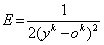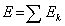Application of Artificial Intelligence Methods in Quantitative Analysis of Gamma-ray Spectra | OMICS International
Journal of Applied & Computational Mathematics

# Application of Artificial Intelligence Methods in Quantitative Analysis of Gamma-ray Spectra

Medhat ME* and Hafiez AA

Experimental Nuclear Physics Department, Nuclear Research Center, Cairo, Egypt

*Corresponding Author:
Medhat ME
Experimental Nuclear Physics Department
Nuclear Research Center
Cairo, Egypt
Tel: 8613693344534
E-mail:[email protected]

Received August 17, 2015; Accepted September 07, 2015; Published September 11, 2015

Citation: Medhat ME, Hafiez AA (2015) Application of Artificial Intelligence Methods in Quantitative Analysis of Gamma-ray Spectra. J Appl Computat Math 4:252. doi:10.4172/2168-9679.1000252

Copyright: © 2015 Medhat ME, et al. This is an open-access article distributed under the terms of the Creative Commons Attribution License, which permits unrestricted use, distribution, and reproduction in any medium, provided the original author and source are credited.

Visit for more related articles at Journal of Applied & Computational Mathematics

#### Abstract

An artificial neural network (ANN) has been trained with real-sample spectra of radioactive materials. Following the training stage ANN was applied to a subset of similar samples Results obtained with the ANN method are in good agreement with results obtained from traditional techniques, showing the high potentiality of ANN.

#### Keywords

Neural network; Radioactivity; Human brain activity; Topology

#### Introduction

Artificial neural network is a form of artificial intelligence which attempt to mimic the behavior of the human brain and nervous system. ANN is composed of an input layer, hidden layers or no hidden layer and an output layer. Each layer includes neurons which are connected to all the neurons of a successive layer. Developments from artificial intelligence like artificial neural networks, ANN, can be regarded as an engineering procedure emulating the human brain activity. The fundamentals of the ANN technique have been described elsewhere [1,2] and several commercial and free codes are accessible nowadays.

Applications of ANN to atomic and nuclear physics has increased during the last two decades mainly in problems related to nuclear reactors. There are several applications of ANN in nuclear spectroscopy e.g.: alpha spectroscopy, peak to background ratio and resolution improvement of detectors employing pulse-shape treatment. ANN has been applied also in gamma-ray spectrometry for automatic identification of radionuclides [3-13].

In the present work we apply ANN for quantitative analysis of natural gamma-ray sources. These methods will be applied successively on analyzing gamma-ray spectra detected from radioactive samples collected from different sources.

#### Principle of ANN

The net peak area under the gamma peak of interest is currently used to determine the elemental concentration. Here, a slightly different approach has been used based on the fact that ANN application disregards additive constants. Then considering the observation that the background contribution at the peak is rather constant for equal irradiation times, the use of the maximum value at the peak channel as an input in ANN calculations, simplifies data handling and provides equivalent results as those obtained when the full area is used. These values and the already known concentrations were given as an input and output data respectively in the ANN training phase. A feed-forward ANN has been used in the learning phase, directly from concentration data obtained in real time. The ANN was trained with the well-known back propagation algorithm in order to learn first and then reproduce the process in order to obtain the concentration of certain elements in some cement samples. The neural network feeds with input parameters like: the neutron beam flux impinging the sample, mass of the sample, spectral information of the detected γ-ray.

For each element, an ANN with three layers (Figure 1), (input, hidden, output) was used to generate the mapping of the p-input parameters (in this work p = 4 to the q-output parameters, here represented by the concentration of the element in the sample (q = 1). The mapping is carried out using the weights of the neuron connections, computed in the supervised training process.

For training, the method for least square error back propagation, the most often used supervised learning algorithm, was used. Supervised learning means that for each example, both the input and the expected output (the output that ANN must produce) are known; so, training the ANN means that the computed outputs must be the closest to the expected outputs for each example. From a general point of view we consider a neural network with p-inputs, one hidden layer with L-neurons (L = 4 in this case) and one output node. The value of the error Ek on an input/output training pattern (xk,yk) is defined by(1)

where ok is the computed output generated when the k-th input vector (x1k … xpk) is presented to the network and the transformation is conducted by the transfer sigmoid function and where yk is the expected value for the output corresponding to input xk. The overall measure of the error is(2)

The rule for changing weights following presentation of input/ output pair k is given by the gradient descent method, i.e. the quadratic error function is minimized by using the iteration process. After the training phase was completed, a test procedure to verify that the neural net effectively did learn the lessons was performed. To train the ANN, first the initial weights are fixed in a random way. Then each sample (the 4 input values) is introduced in the ANN, which compute the output (Ok in the above equations), it is say, the actual concentration value. This value is compared with expected concentration value and according to the error, the weights are modified so that both values (computed concentration and expected concentration) become the closest possible.

Usually the training set is defined experimentally. In this case due to the rather low number of available samples, according to the experience of the authors training set of 5 spectra randomly selected and a testing set of three spectra were used in each ANN. Results confirmed that this selection was adequate.

An ANN for each the detected elements, similar topology, was trained in an independent manner and the sigmoid activation function was used in the hidden and output layers. The topology and the parameters used (learning rate, momentum, error value, etc.) were obtained in an experimental way.

#### Results and Discussion

Without missing generality in this ANN application only the major elements observed in the spectra were considered. Trace elements, although important for other studies, were not statistically useful for the present purpose. Elemental concentrations were calculated by standard traditional methods methodology in the way described above. These results were considered as ‘‘expected concentrations’’. As for traditional methods uncertainties, overall values ranged between 3% and 12%. A feed-forward neural network, with definite topology was independently trained in parallel with the backward error propagator to determine the elemental concentrations of interest in the samples of this study. Spectra were randomly selected and used in training the network and three were used in testing it. All training cases were learned correctly by each of the six neural networks. The agreement is illustrated in Figure 2, where concentrations are shown for each element in samples. To assess the goodness of the ANN method in providing concentrations values, a comparison with traditional methods results was done and is shown in Figure 3. A linear regression calculation for results from all elements, in the test and training sets, was performed. Correlations factor, r2, of 0.86 and 0.99 were obtained for the test and training sets, respectively (Figures 2 and 3).

Figure 2: Elemental concentration obtained from the three samples of the test set using ANN (CNN) and traditional methods (Cexp), are shown for three cement samples respectively for each element.

Figure 3: The predicted concentrations from ANN (CNN) as compared with traditional methods (Cexp) concentrations.

#### Conclusion

The main purpose of this work was to explore the application of ANN for a fast analysis of traditional methods in a study of elemental concentration of some cement samples. It has been proved that this methodology provides results as well as those obtained by one of the already accepted methods. Even though the number of cases was rather limited, the decision to use independent neuronal topologies for each element working in parallel provided reliable results. Surely, the experimental results would be improved with a greater number of cases to use as training and test sets. So, the use of ANN as a reasonable alternative for the elemental concentration determination in NAA procedure has been established. In addition, this ANN application proved to be fast enough as to predict that the analysis of a great number of samples can be accomplished in few minutes, avoiding the direct participation of the analyst in each one. Once the ANN has been trained, its use become fast and thus permitting the automation of NAA spectra analysis and reducing costs and time.

#### References

Select your language of interest to view the total content in your interested language

### Article Usage

• Total views: 12816
• [From(publication date):
October-2015 - Dec 15, 2019]
• Breakdown by view type
• HTML page views : 8955Can't read the image? click here to refresh